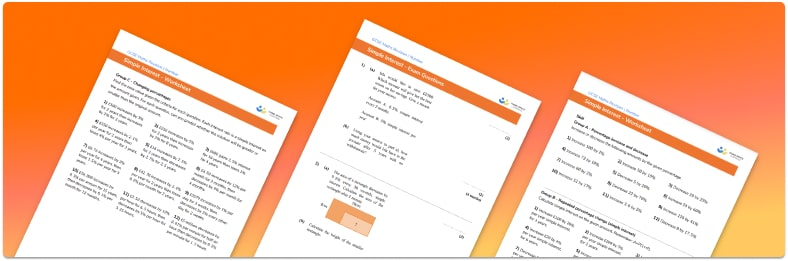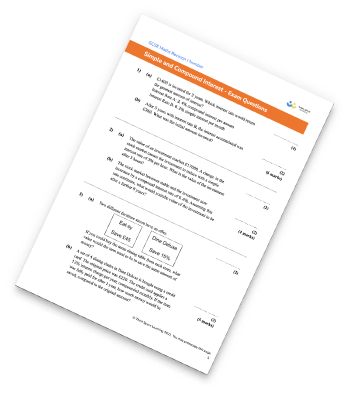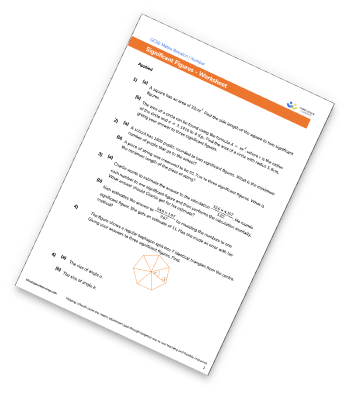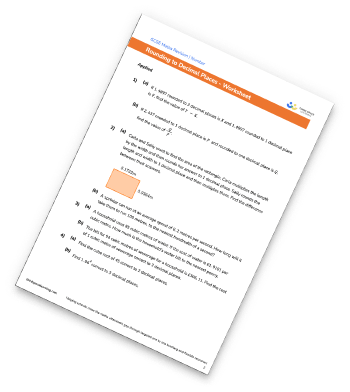# Simple Interest WorksheetHelp your students prepare for their Maths GCSE with this free simple interest worksheet of 44 questions and answers

• Section 1 of the simple interest worksheet contains 36 skills-based simple interest questions, in 3 groups to support differentiation
• Section 2 contains 4 applied simple interest questions with a mix of word problems and deeper problem solving questions
• Section 3 contains 4 foundation and higher level GCSE exam style simple interest questions
• Answers and a mark scheme for all simple interest questions are provided
• Questions follow variation theory with plenty of opportunities for students to work independently at their own level
• All questions and math worksheets created by fully qualified expert secondary maths teachers
• Suitable for GCSE maths revision for AQA, OCR and Edexcel exam boards
• To receive this resource and regular emails with more free resources, blog posts and other Third Space updates, enter your email address and click below.

• This field is for validation purposes and should be left unchanged.

You can unsubscribe at any time (each email we send will contain an easy way to unsubscribe). To find out more about how we use your data, see our privacy policy.

### Simple interest at a glance

Simple interest is a method of calculating interest based on what is in the account at the beginning of the period. Simple interest could be used to calculate the amount of interest payable into a bank account or on a loan.

Calculating simple interest is done using the annual interest rate. The percentage of the original value is calculated and that amount of money is added to the account each year. For example, if £100 is invested into a savings account with a simple interest rate of 4% per annum, then £4 is added to the account each year. The total amount of interest will be equal to £4 multiplied by the number of years that the money is invested for.

There is a simple interest formula which can be used to calculate the amount of simple interest to be paid: Simple interest = P x R x T where P is the principal (original) investment, R is the interest rate (as a decimal) and T is time.

Looking forward, students can then progress to additional number worksheets, for example aor aFor more teaching and learning support on Number our GCSE maths lessons provide step by step support for all GCSE maths concepts.

## Related worksheets

Simple And Compound Interest WorksheetSignificant Figures WorksheetRounding To The Nearest 10, 100, And 1000 WorksheetRounding Decimals Worksheet## Do you have KS4 students who need more focused attention to succeed at GCSE?There will be students in your class who require individual attention to help them succeed in their maths GCSEs. In a class of 30, it’s not always easy to provide.

Help your students feel confident with exam-style questions and the strategies they’ll need to answer them correctly with our dedicated GCSE maths revision programme.

Lessons are selected to provide support where each student needs it most, and specially-trained GCSE maths tutors adapt the pitch and pace of each lesson. This ensures a personalised revision programme that raises grades and boosts confidence.

Find out more